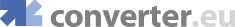online mass flux density conversions, gram/second square centimeter, gram/second square meter
Engineering converters
Heat converters
Fluids converters
 Concentr. Liquid Solution Concentration - Molar Flow Flow - Mass Flow - Molar Mass Flux Density Permeability Surface Tension Viscosity - Dynamic Viscosity - Kinematic
Light converters
Electricity converters
Magnetism converters
Other converters

 Show currency exchange rate: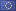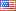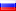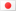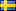… Get a Converter API tool for your website for free!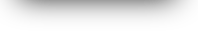All Mass Flux Density Conversion
Convert fromConvert to
g/s∙cm² =  g/s∙cm²
 Gram/Second Square Centimeter g/s∙cm² Gram/Second Square Meter g/s∙m² Kilogram/Hour Square Foot kg/h∙ft² Kilogram/Hour Square Meter kg/h∙m² Kilogram/Second Square Meter kg/s∙m² Pound/Hour Square Foot lb/h∙ft² Pound/Second Square Foot lb/s∙ft²

 Gram/Second Square Centimeter g/s∙cm² Gram/Second Square Meter g/s∙m² Kilogram/Hour Square Foot kg/h∙ft² Kilogram/Hour Square Meter kg/h∙m² Kilogram/Second Square Meter kg/s∙m² Pound/Hour Square Foot lb/h∙ft² Pound/Second Square Foot lb/s∙ft²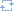Change directionMost popular convertion pairs of mass flux density

© converter.eu  |  API  |  feedback